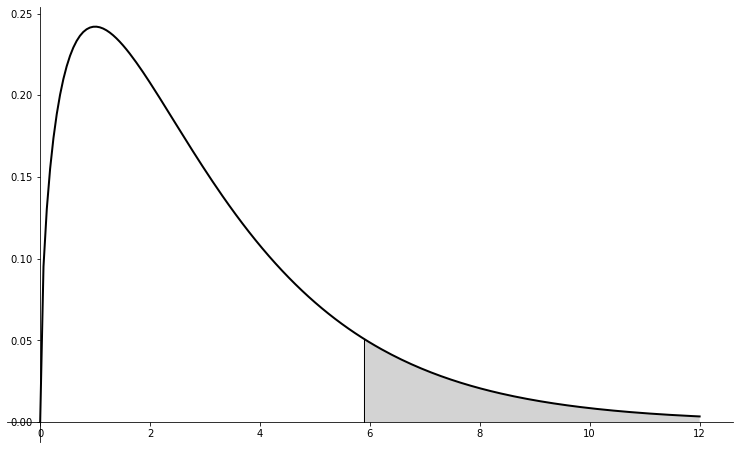# The $\chi^2$-Test¶

Recently, we've been discussing relationships between variables. For example, linear regression examines the relationship between two numerical variables. Similarly, the $\chi^2$-test examines the relationship between categorical variables.

This is covered in sections 6.3 and 6.4 of our text.

## The two types of $\chi^2$ tests¶

As we'll see, there are two somewhat different types of $\chi^2$-tests. Specifically, there's

• the $\chi^2$-test for homogeneity, which tests whether frequency counts for a single categorical variable are distributed similarly across different populations and
• the $\chi^2$-test for independence, which tests whether there is a significant association between two categorical variables from a single population.

## A basic example testing homogeneity¶

We'll start with an important, concrete question taken right from our text: Is a given pool of potential jurors in a county racially representative of that county?

### Specific data¶

Here's some specific data representing 275 jurors in a small county. Jurors identified their racial group, as shown in the table below. We would like to determine if these jurors are racially representative of the population.

Race White Black Hispanic Other Total
Representation in juries 205 26 25 19 275
Percentages for registered voters 0.72 0.07 0.12 0.09 1.00
Expected count 198 19.25 33 24.75 275

### Using Python's chisquare¶

Python's scipy.stats module has a chisquare function built for exactly this situation and it's pretty easy to use:

from scipy.stats import chisquare
chisquare([205, 26, 25, 19], f_exp = [198.0, 19.25, 33.0, 24.75])

Power_divergenceResult(statistic=5.8896103896103895, pvalue=0.11710619130850619)

There's a lot going on in the background here but, ultimately, we are interested in that $p$-value. If we are looking for a 95% confidence level, then we are unable to reject the null hypothesis here, in spite of the deviation from expected counts that we see in the data.

### Some formulae¶

The $p$-value is computed using the $\chi^2$ statistic, which we find as follows:

We suppose that we are to evaluate whether there is convincing evidence that a set of observed counts $O_1$, $O_2$, ..., $O_k$ in $k$ categories are unusually different from what might be expected under a null hypothesis. Call the \emph{expected counts} that are based on the null hypothesis $E_1$, $E_2$, ..., $E_k$. If each expected count is at least 5 and the null hypothesis is true, then the test statistic below follows a chi-square distribution with $k-1$ degrees of freedom: $$\chi^2 = \frac{(O_1 - E_1)^2}{E_1} + \frac{(O_2 - E_2)^2}{E_2} + \cdots + \frac{(O_k - E_k)^2}{E_k}$$

### Computation on the computer¶

ch_sq = ((205-198)**2/198 + (26-19.25)**2/19.25 +(25-33)**2/33 + (19-24.75)**2/24.75)
ch_sq

5.8896103896103895

## The p-value¶

The $p$-value is computed from the test-statistic using a new distribution, called the F-distribution. Geometrically, it represents the area under the curve below and to the right of $5.88$:## An example testing independence¶

Sometimes, we have two categorical variables and we want to know if they are independent or not. This is also called the Chi-Square test for homogeneity.

### Example¶

Here's an example from the R-Tutorial examining whether exercise and smoking are independent of one another. We'll use the following data:

Smokes/Exercises Frequently Some None
Never 435 420 90
Occasionally 60 20 15
Regularly 45 35 5
Heavily 35 15 5

The rows indicate how much the participant smokes and the columns indicate how much they exercise. Our null hypothesis is that these are independent; our alternative hypothesis is contrary.

### Doing it with Python¶

Python's scipy.stats module has another command called chi2_contingency built for this situation. We can enter a small table like this into Python and get the $p$-value with chi2_contingency as follows:

from scipy.stats import chi2_contingency
A = [
[435,420,90],
[60,20,15],
[45,35,5],
[35,15,5]
]
chi2_contingency(A)

0.00011960587467155845

It looks like we reject the null hypothesis of independence.